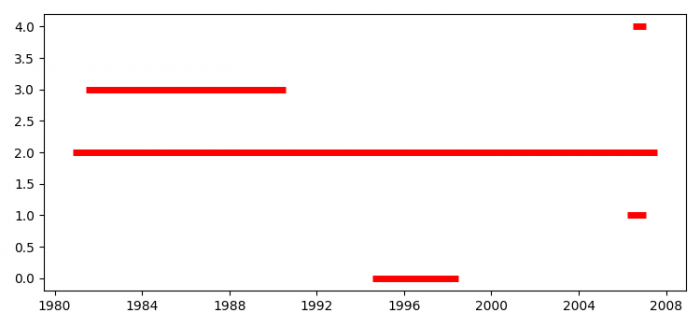# How to plot a stacked event duration using Python Pandas?

To plot a stacked event duration using Python Pandas, we can take the following steps

• Set the figure size and adjust the padding between and around the subplots.
• Create a dataframe with lists of xmin and its corresponding xmax.
• Use hlines() method to plot a stacked event duration.
• To display the figure, use show() method.

## Example

import pandas as pd
from datetime import datetime as dt
from matplotlib import pyplot as plt
plt.rcParams["figure.figsize"] = [7.50, 3.50]
plt.rcParams["figure.autolayout"] = True
df = pd.DataFrame(dict(xmin=[dt.strptime('1994-07-19', '%Y-%m-%d'),
dt.strptime('2006-03-16', '%Y-%m-%d'),
dt.strptime('1980-10-31', '%Y-%m-%d'),
dt.strptime('1981-06-11', '%Y-%m-%d'),
dt.strptime('2006-06-28', '%Y-%m-%d')],
xmax=[dt.strptime('1998-06-30', '%Y-%m-%d'),
dt.strptime('2007-01-24', '%Y-%m-%d'),
dt.strptime('2007-07-31', '%Y-%m-%d'),
dt.strptime('1990-08-01', '%Y-%m-%d'),
dt.strptime('2007-01-24', '%Y-%m-%d')]
))
plt.hlines(df.index, df.xmin, df.xmax, lw=5, colors='red')
plt.show()

## Output